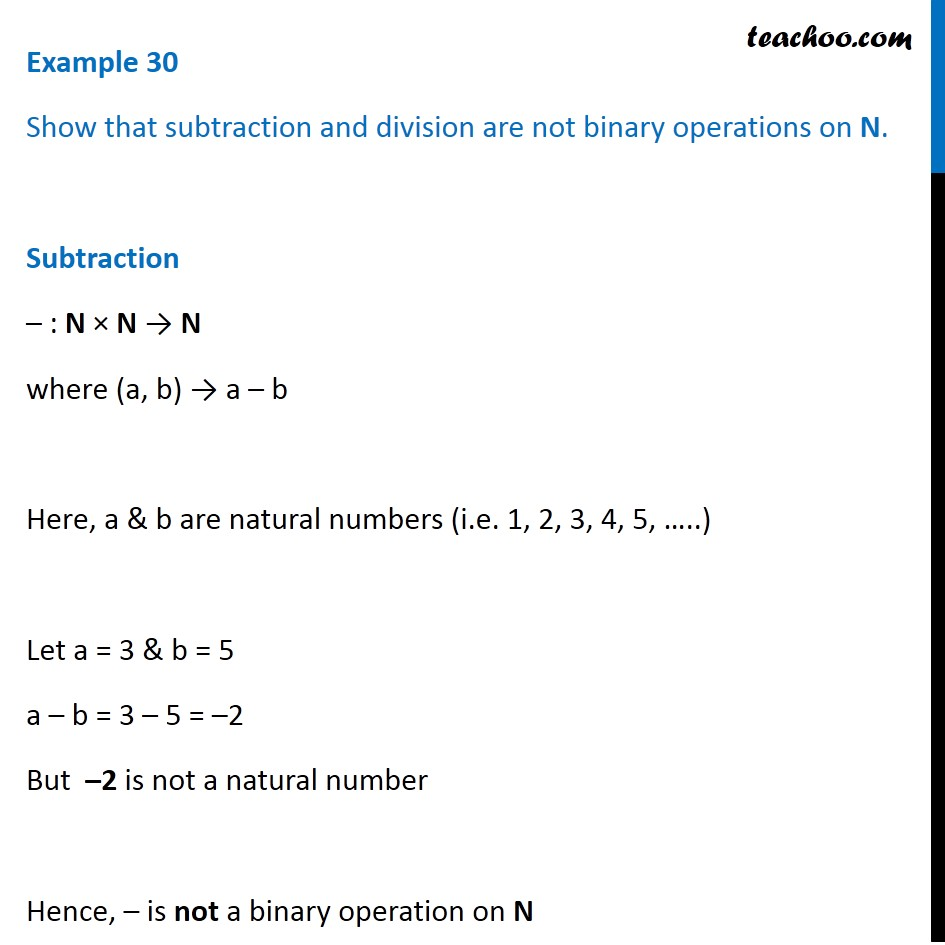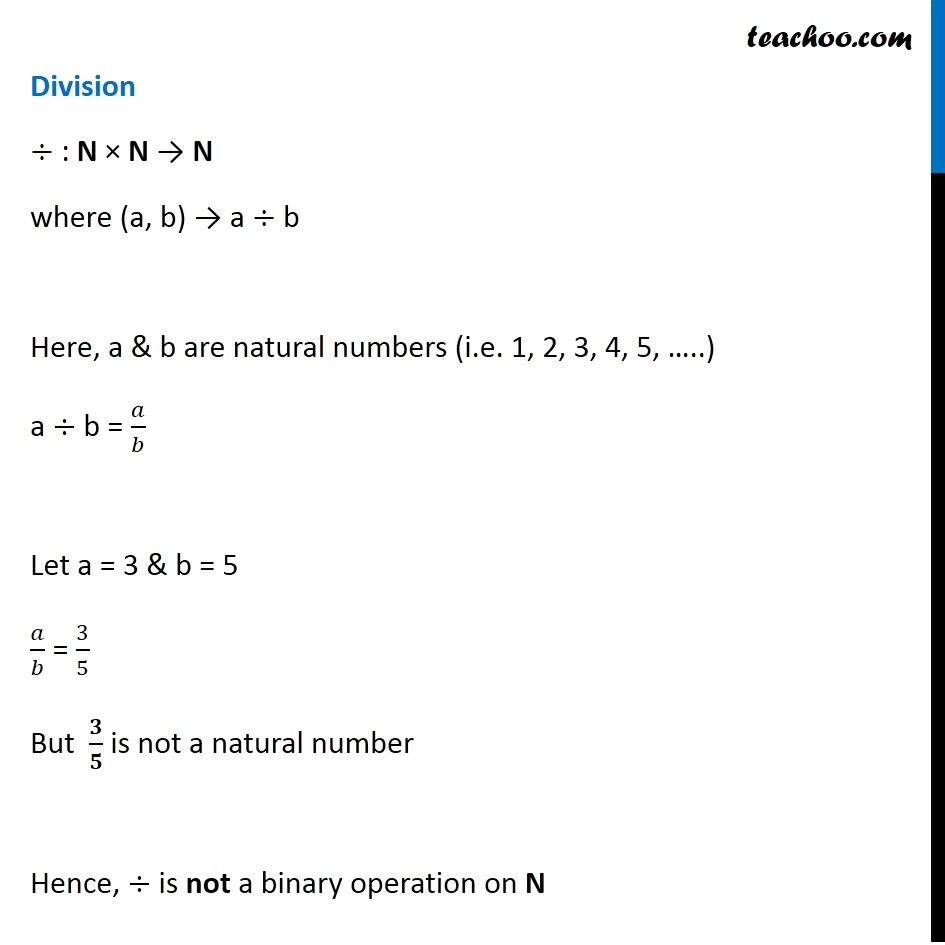Examples

Chapter 1 Class 12 Relation and Functions
Serial order wiseLearn in your speed, with individual attention - Teachoo Maths 1-on-1 Class

### Transcript

Question 13 Show that subtraction and division are not binary operations on N. Subtraction – : N × N → N where (a, b) → a – b Here, a & b are natural numbers (i.e. 1, 2, 3, 4, 5, …..) Let a = 3 & b = 5 a – b = 3 – 5 = –2 But –2 is not a natural number Hence, – is not a binary operation on N Division ÷ : N × N → N where (a, b) → a ÷ b Here, a & b are natural numbers (i.e. 1, 2, 3, 4, 5, …..) a ÷ b = 𝑎/𝑏 Let a = 3 & b = 5 𝑎/𝑏 = 3/5 But 𝟑/𝟓 is not a natural number Hence, ÷ is not a binary operation on N